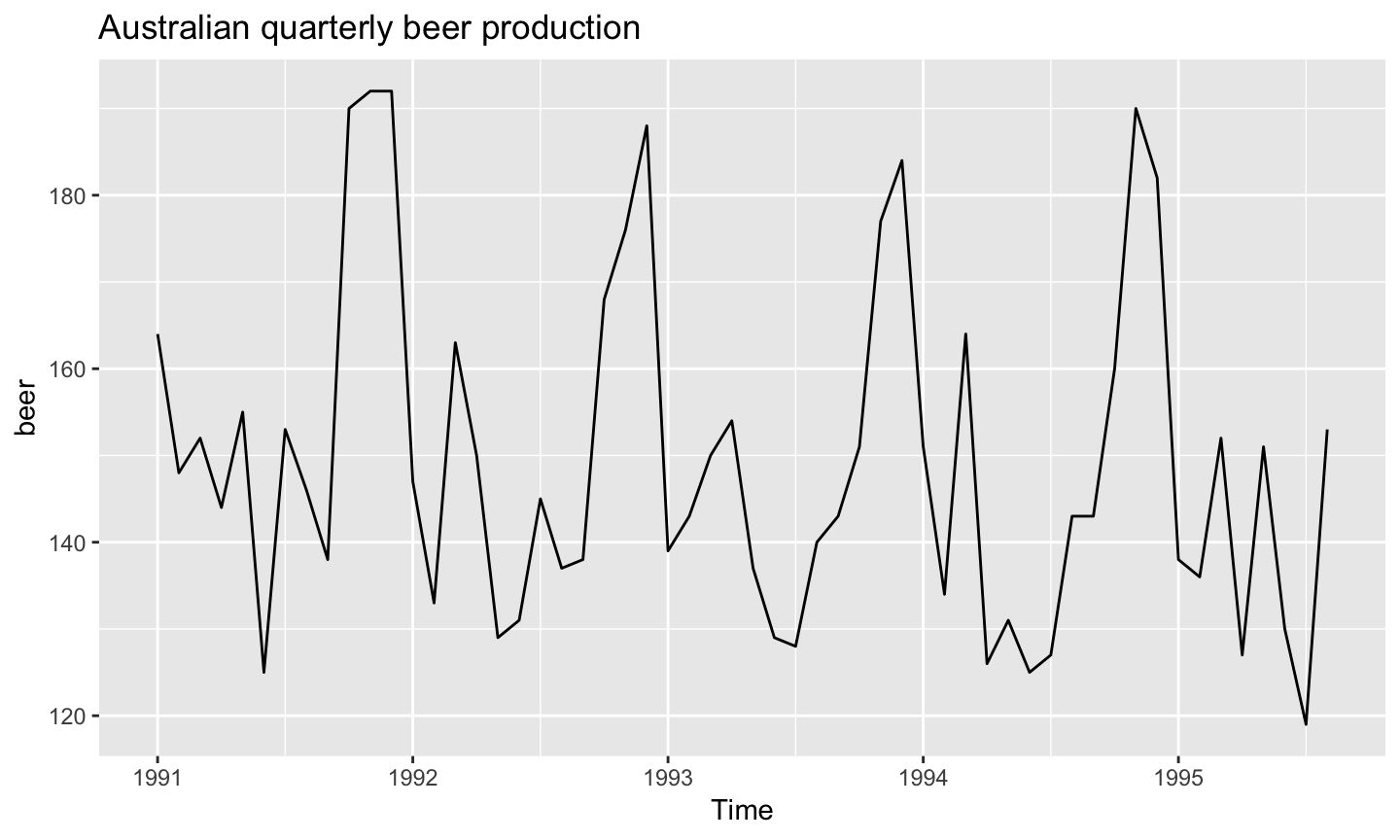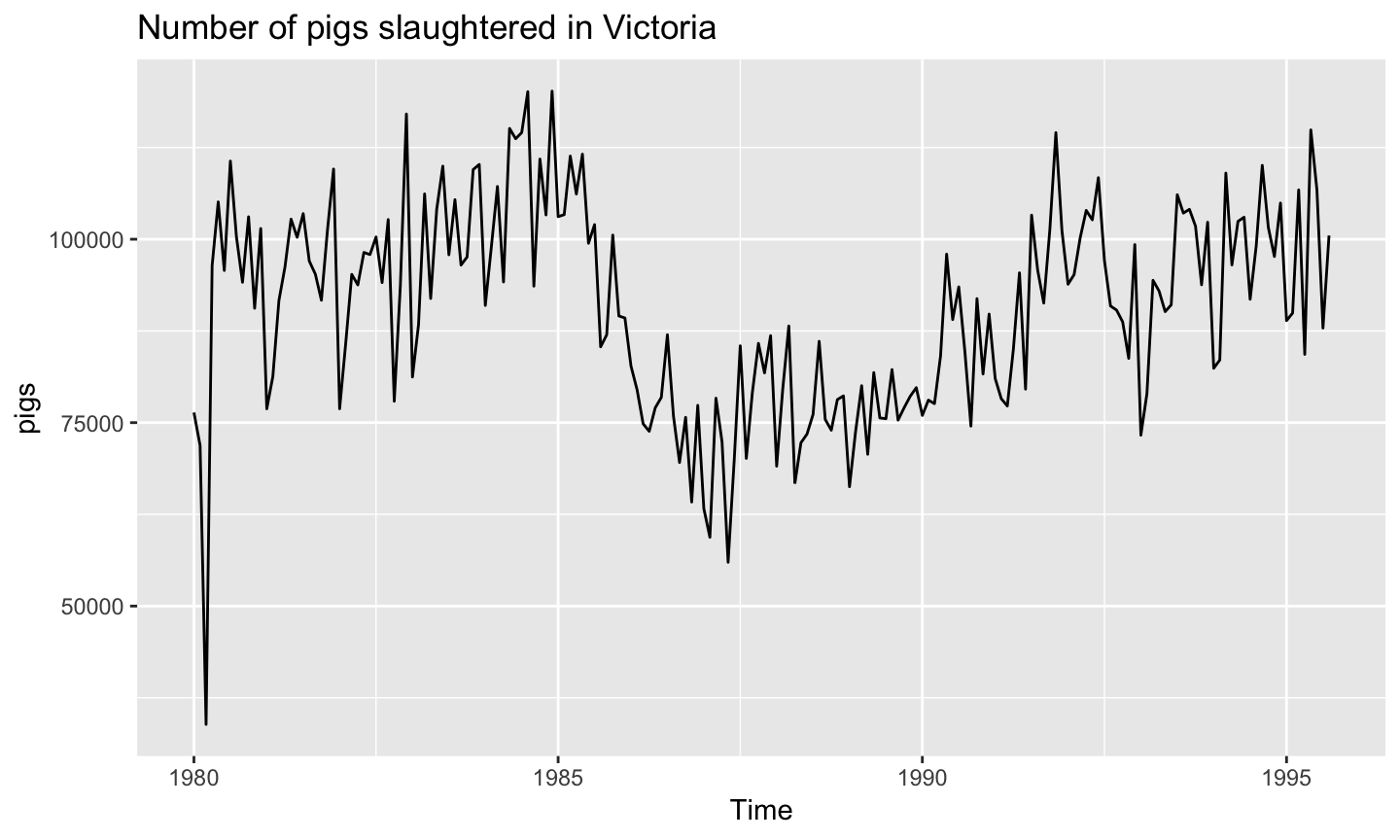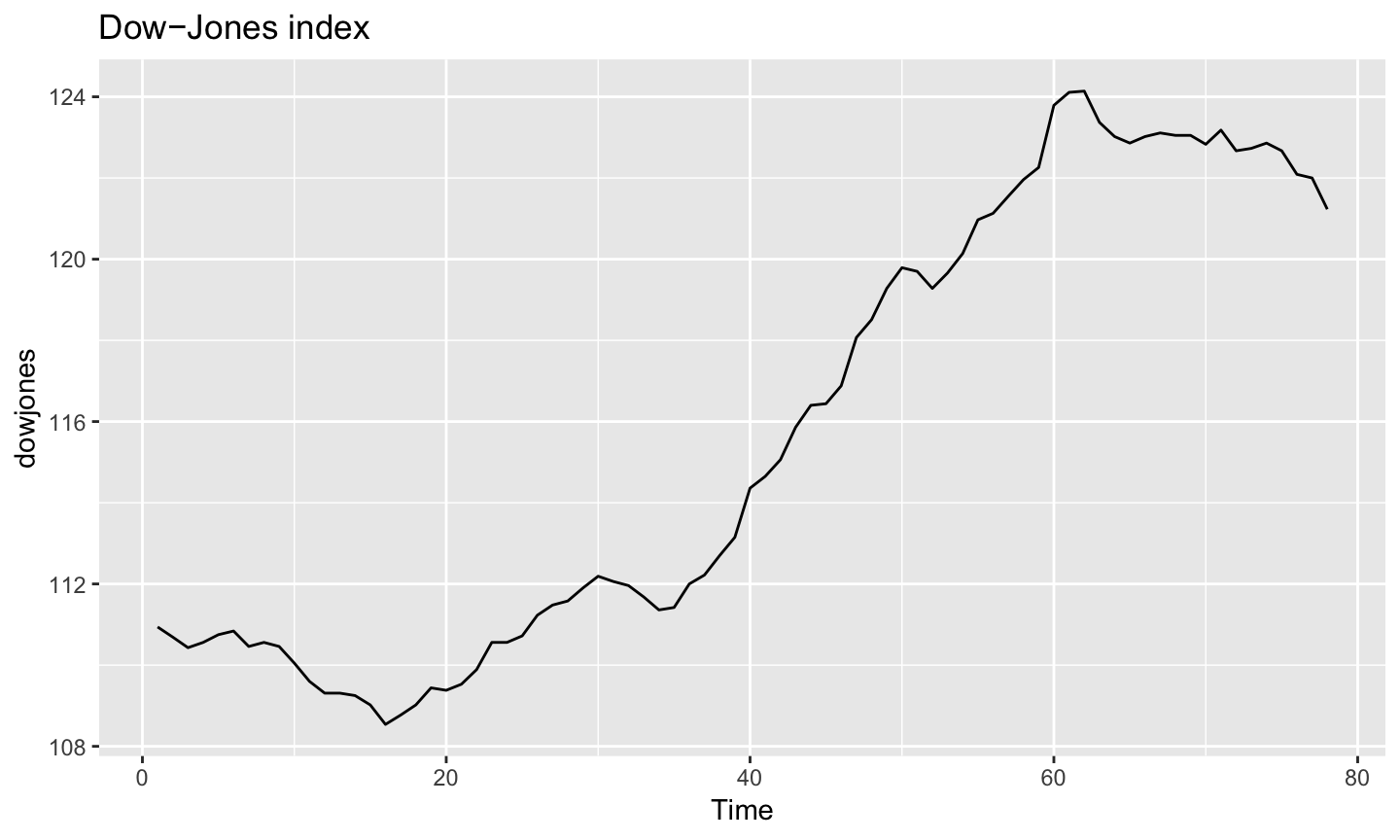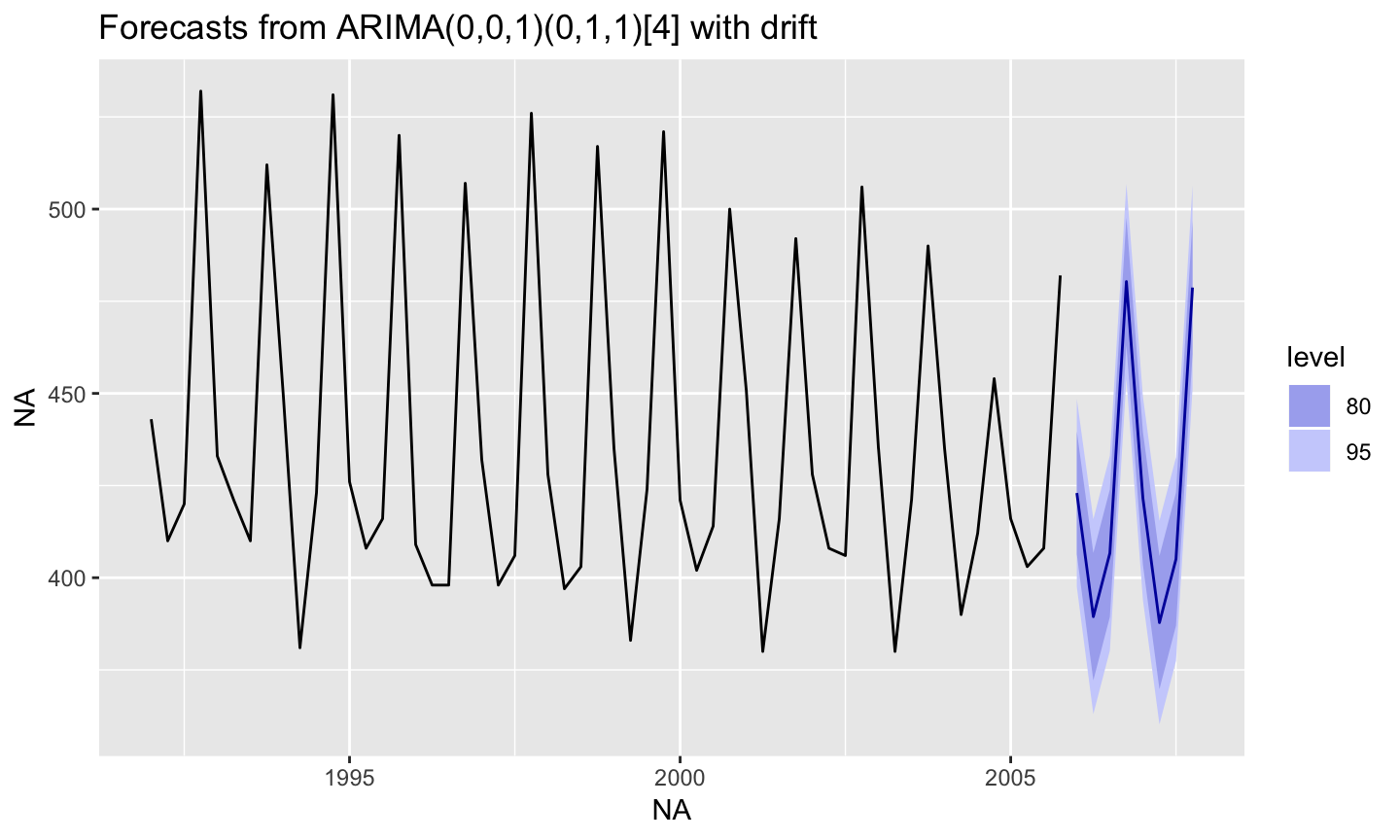School of Economics and Management
Beihang University
http://yanfei.site

## In this lecture we are going to learn…

• Some basic forecasting methods
• Forecasting time series with complex seasonality
• Dealing with missing values and outliers

## Examples of time series

```library(fpp2)
library(ggplot2)
library(forecast)

autoplot(beer, main = 'Australian quarterly beer production')````autoplot(pigs, main = 'Number of pigs slaughtered in Victoria')``autoplot(dowjones, main = 'Dow−Jones index')`## Forecasting

• Forecasting is estimating how the sequence of observations will continue into the future.
• We usually think probabilistically about future sample paths
• What range of values covers the possible sample paths with 80% probability?
```beer <-  window(ausbeer, start = 1992, end = c(2005,4))
beer.arima <- auto.arima(beer)
autoplot(forecast(beer.arima))```## Some basic forecasting methods

### Very simple forecasting methods

1. Average method: forecast of all future values is equal to mean of historical data.

2. Naïve method: forecasts equal to last observed value.

3. Seasonal naïve method: forecasts equal to last value from same season.

```## average method
beerfit.mean <- meanf(beer, h = 11)

## naive method
beerfit.naive <- naive(beer, h = 11)

## seasonal naive method
beerfit.snaive <- snaive(beer, h = 11)

cols <- c("mean" = "#0000ee","naive" = "#ee0000","snaive" = "green")

## plot
autoplot(beerfit.mean, PI = FALSE, main ='Forecasts for quarterly beer production') +
geom_line(aes(x=time(beerfit.mean\$mean),y=beerfit.mean\$mean,colour='mean')) +
geom_line(aes(x=time(beerfit.naive\$mean),y=beerfit.naive\$mean,colour='naive')) +
geom_line(aes(x=time(beerfit.snaive\$mean),y=beerfit.snaive\$mean,colour='snaive')) +
guides(fill=FALSE) +
scale_colour_manual(name="Forecasts",values=cols)```